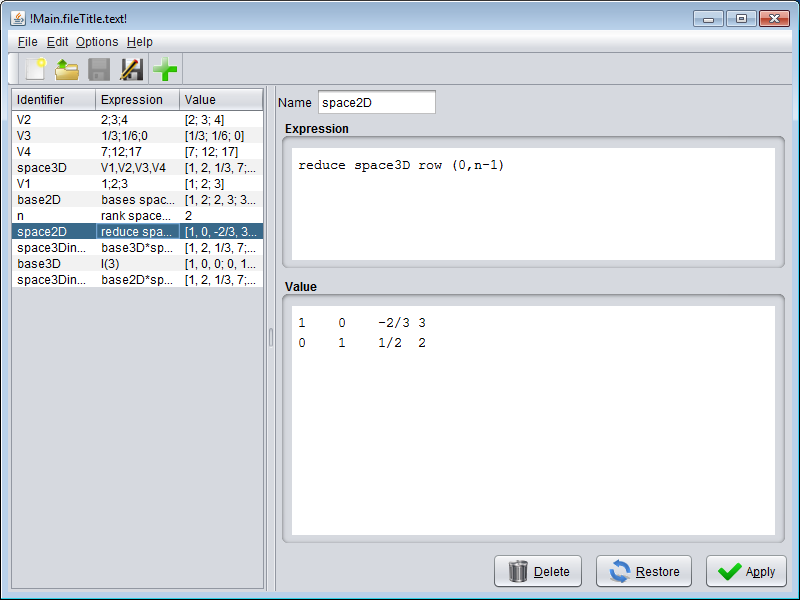View on GitHub

# Ratio

## Rational matrix calculator

Ratio is a java application that handles rational numbers and matrices.

Evaluating expressions based on rational numbers and matrices are going to get easy. For example you can compute the product between a matrix and a vector as

`````` 1  2  3     1       3
2  3  4  x  1/2  =  29/6
3  2  1     1/3     203
``````

typing simply

``````(1, 2, 3; 2, 3, 4; 3, 4, 5) * (1; 1/2; 1/3)
``````

Most of the algebraic operators are available, such `+`, `-`, `*`, `/`, operators of composition of matrices and the general functions on matrices such as rank, inverse, determinant, reduction, identity and calculation of basis vectors.

To get details information you can check out the https://github.com/m-marini/ratio/wiki.

To run Ratio you need the Java 1.7 runtime environment installed.